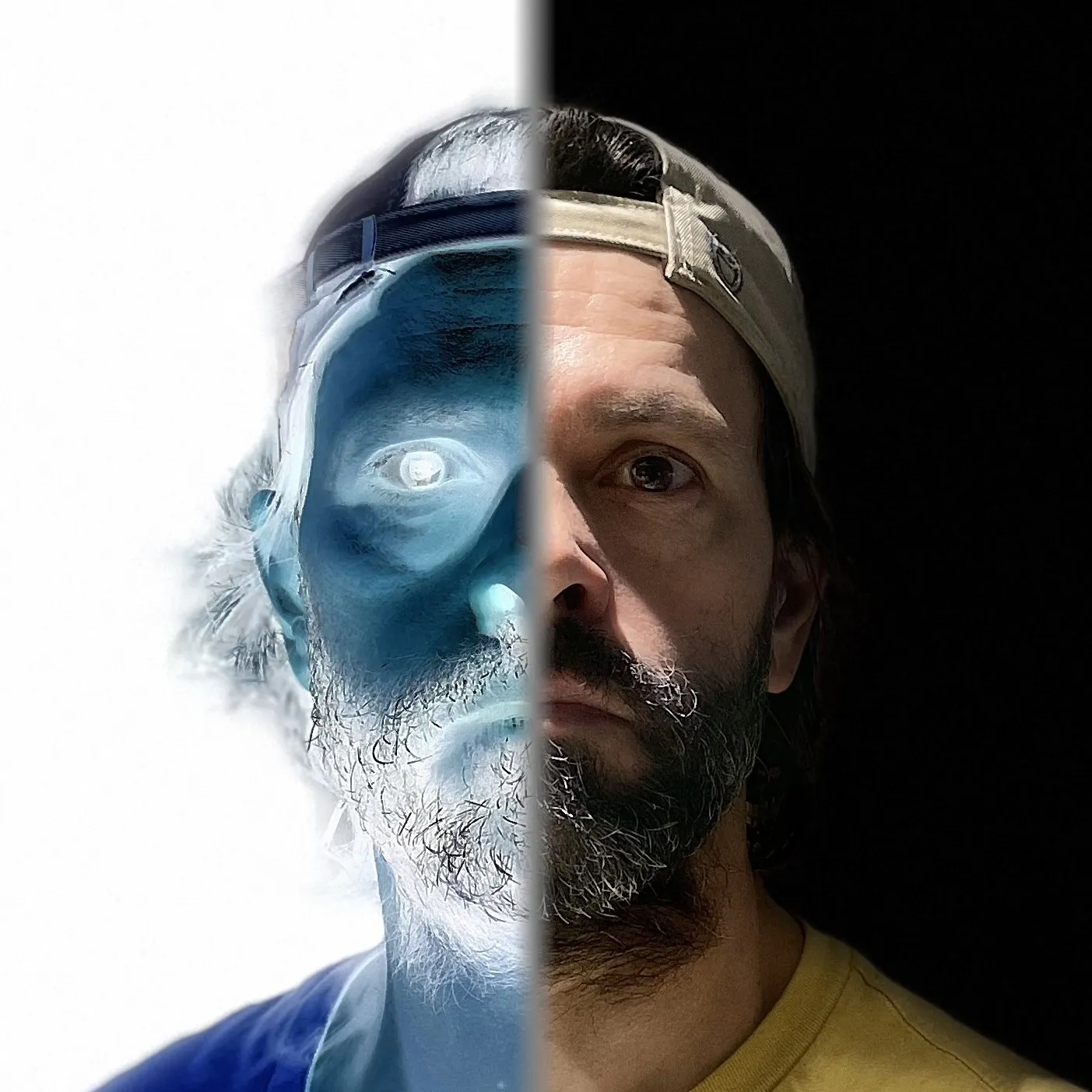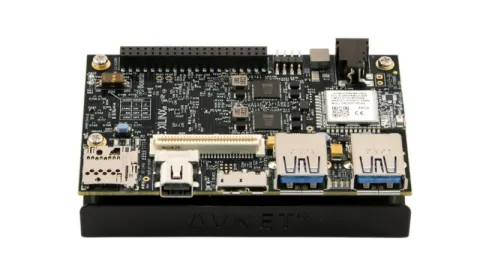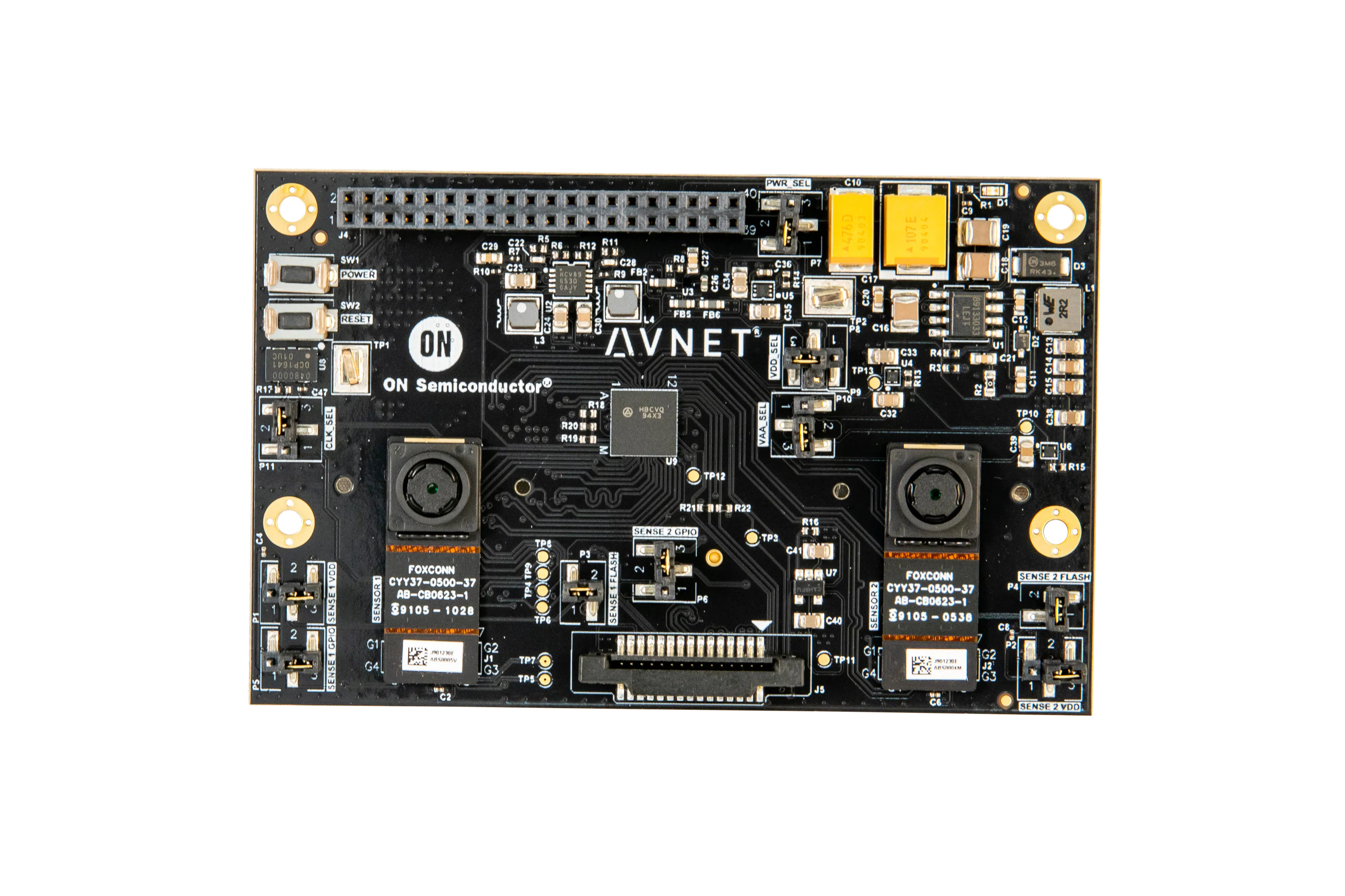Stereo Face Detection with the Dual Camera Mezzanine

This guide provides detailed instructions for implementing face detection on two (2) cameras using the Ultra96-V2 and Dual-Camera Mezzanine.

IntermediateWork in progress6 hours500

Things used in this project

Hardware componentsAvnet Ultra96-V2
×1
 DisplayPort monitor
×1Avnet 96Boards ON Semiconductor Dual Camera Mezzanine
×1

Code

u96v2_sbc_dualcam_ar0144_stereo_face_detection.py

Python
Stereo Face Detection
'''

you may not use this file except in compliance with the License.
You may obtain a copy of the License at

Unless required by applicable law or agreed to in writing, software
WITHOUT WARRANTIES OR CONDITIONS OF ANY KIND, either express or implied.
See the License for the specific language governing permissions and
'''

# USAGE
# python sterep_face_detection.py [--input 0] [--detthreshold 0.55] [--nmsthreshold 0.35]

from ctypes import *
from typing import List
import cv2
import numpy as np
import vart
import pathlib
import xir
import os
import math
import time
import sys
import argparse

sys.path.append(os.path.abspath('../'))
sys.path.append(os.path.abspath('./'))
from vitis_ai_vart.facedetect import FaceDetect
from vitis_ai_vart.utils import get_child_subgraph_dpu

# construct the argument parse and parse the arguments
ap = argparse.ArgumentParser()
help = "input camera identifier (default = 0)")
help = "face detector softmax threshold (default = 0.55)")
help = "face detector NMS threshold (default = 0.35)")
args = vars(ap.parse_args())

if not args.get("input",False):
inputId = 0
else:
inputId = int(args["input"])
print('[INFO] input camera identifier = ',inputId)

if not args.get("detthreshold",False):
detThreshold = 0.55
else:
detThreshold = float(args["detthreshold"])
print('[INFO] face detector - softmax threshold = ',detThreshold)

if not args.get("nmsthreshold",False):
nmsThreshold = 0.35
else:
nmsThreshold = float(args["nmsthreshold"])
print('[INFO] face detector - NMS threshold = ',nmsThreshold)

# Initialize Vitis-AI/DPU based face detector
densebox_xmodel = "/usr/share/vitis_ai_library/models/densebox_640_360/densebox_640_360.xmodel"
densebox_graph = xir.Graph.deserialize(densebox_xmodel)
densebox_subgraphs = get_child_subgraph_dpu(densebox_graph)
assert len(densebox_subgraphs) == 1 # only one DPU kernel
densebox_dpu = vart.Runner.create_runner(densebox_subgraphs,"run")
dpu_face_detector = FaceDetect(densebox_dpu,detThreshold,nmsThreshold)
dpu_face_detector.start()

# Initialize the camera input
print("[INFO] starting camera input ...")
cam = cv2.VideoCapture(inputId)
cam.set(cv2.CAP_PROP_FRAME_WIDTH,640)
cam.set(cv2.CAP_PROP_FRAME_HEIGHT,480)
if not (cam.isOpened()):
print("[ERROR] Failed to open camera ", inputId )
exit()

# Get width and height of video stream
w2 = int(cam.get(cv2.CAP_PROP_FRAME_WIDTH))
h = int(cam.get(cv2.CAP_PROP_FRAME_HEIGHT))
print("[INFO] camera size =",w2,"X",h)
w = int(w2/2)
print("[INFO] half size =",w,"X",h)

# inspired from cvzone.Utils.py
def cornerRect( img, bbox, l=20, t=5, rt=1, colorR=(255,0,255), colorC=(0,255,0)):

#x1,y1,w,h = bbox
#x2,y2 = x+w, y+h
x1,y1,x2,y2 = bbox
x1 = int(x1)
x2 = int(x2)
y1 = int(y1)
y2 = int(y2)

if rt != 0:
cv2.rectangle(img,(x1,y1),(x2,y2),colorR,rt)

# Top Left x1,y1
cv2.line(img, (x1,y1), (x1+l,y1), colorC, t)
cv2.line(img, (x1,y1), (x1,y1+l), colorC, t)
# Top Right x2,y1
cv2.line(img, (x2,y1), (x2-l,y1), colorC, t)
cv2.line(img, (x2,y1), (x2,y1+l), colorC, t)
# Top Left x1,y2
cv2.line(img, (x1,y2), (x1+l,y2), colorC, t)
cv2.line(img, (x1,y2), (x1,y2-l), colorC, t)
# Top Left x2,y2
cv2.line(img, (x2,y2), (x2-l,y2), colorC, t)
cv2.line(img, (x2,y2), (x2,y2-l), colorC, t)

return img

# loop over the frames from the video stream
while True:
# Capture image from camera

# Extract left/right images and resize
right_frame = frame[:,1:w+1,:]
right_frame = cv2.resize(right_frame,(w2,h))
left_frame = frame[:,w:w2+1,:]
left_frame = cv2.resize(left_frame,(w2,h))

# Make copies of left/right images for graphical annotations and display
frame1 = left_frame.copy()
frame2 = right_frame.copy()

# Vitis-AI/DPU based face detector
left_faces = dpu_face_detector.process(left_frame)
right_faces = dpu_face_detector.process(right_frame)

# if one face detected in each image, calculate the centroids to detect distance range
distance_valid = False
if (len(left_faces) == 1) & (len(right_faces) == 1):

# loop over the left faces
for i,(left,top,right,bottom) in enumerate(left_faces):
left_cx = int((left+right)/2)
left_cy = int((top+bottom)/2)
cornerRect(frame2,(left,top,right,bottom),colorR=(255,255,255),colorC=(255,255,255))
cv2.circle(frame2,(left_cx,left_cy),4,(255,255,255),-1)

# loop over the right faces
for i,(left,top,right,bottom) in enumerate(right_faces):
right_cx = int((left+right)/2)
right_cy = int((top+bottom)/2)
cornerRect(frame2,(left,top,right,bottom),colorR=(255,255,0),colorC=(255,255,0))
cv2.circle(frame2,(right_cx,right_cy),4,(255,255,0),-1)

delta_cx = abs(left_cx - right)
delta_cy = abs(right_cy - left_cy)
message = "delta_cx="+str(delta_cx)
#print(message)
cv2.putText(frame2,message,(20,20),cv2.FONT_HERSHEY_SIMPLEX,0.75,(255,255,0),2)

if ( (delta_cx > 15) & (delta_cx < 25) ):
distance_valid = True

# loop over the left faces
for i,(left,top,right,bottom) in enumerate(left_faces):

if distance_valid == True:
cornerRect(frame1,(left,top,right,bottom),colorR=(0,255,0),colorC=(0,255,0))
if distance_valid == False:
cornerRect(frame1,(left,top,right,bottom),colorR=(0,0,255),colorC=(0,0,255))

# Display the processed image
display_frame = cv2.hconcat([frame1, frame2])
cv2.imshow("Stereo Face Detection", display_frame)
key = cv2.waitKey(1) & 0xFF

# if the `q` key was pressed, break from the loop
if key == ord("q"):
break

# Stop the face detector
dpu_face_detector.stop()
del densebox_dpu

# Cleanup
cv2.destroyAllWindows()

Credits

Mario Bergeron

18 projects • 139 followers
Mario Bergeron is a Technical Marketing Engineer working at Avnet, specializing in embedded vision and machine learning.